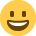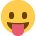Read the community guidelines before posting ✨

# Probability

Posts: 1,876,324 The Mix Honorary Guru
can someone help me with this question please...

Urn 1 has 2 white balls and 4 red balls.
Urn 2 has 2 white balls and 1 red ball.
a ball is then randomly picked from urn 1 and placed into urn 2
what is the probability of picking a white ball from urn 2?

i think ive done it wrong...
there is 0.33 prob that a white ball will be picked from urn 1
if the white ball then goes into urn 2, there is 0.75 prob that a white ball will be picked from urn 2

from this i then multiplied the 2 together 0.33*0.75 and got 0.2475, but this seems wrong because even if a red ball was moved into urn 2, there would be a 0.5 prob that a white was picked from it.

Thanks

• Posts: 1,876,324 The Mix Honorary Guru
Nah, you've got it about right. I did it as 2/6X3/4 and it came out as 1/4. It's the probability of having them BOTH be white, so the probability of it being white in the first one reduces the probability of it being white in the second one.

If that makes sense.
• Posts: 1,876,324 The Mix Honorary Guru
cool, cheers!• Posts: 1,876,324 The Mix Honorary Guru
OK
let W be the probability of pulling a known white ball, R be the probability of picking a known red ball and M that of getting the mystery ball

you know that W+R+M=1

does that help any?
• Posts: 1,876,324 The Mix Honorary Guru
Big Gay wrote: »
OK
let W be the probability of pulling a known white ball, R be the probability of picking a known red ball and M that of getting the mystery ball

you know that W+R+M=1

does that help any?
There is no mystery ball.
• Posts: 1,876,324 The Mix Honorary Guru
big gay franki is right, there is no mystery ball that we need to look at. we are only interested in white balls, so the probabilities that a white ball is picked from each urn is definate, so I think we can just multiply them together (multiplication rule in probability).

xsazx thanks for ur post but not really understanding that one, because we are only interested in a white ball being picked from urn1 so the probability of a red ball being picked is irrelevant is it not?

god i wish they would just give us mark schemes!
• Posts: 1,876,324 The Mix Honorary Guru
big gay franki is right, there is no mystery ball that we need to look at. we are only interested in white balls, so the probabilities that a white ball is picked from each urn is definate, so I think we can just multiply them together (multiplication rule in probability).

xsazx thanks for ur post but not really understanding that one, because we are only interested in a white ball being picked from urn1 so the probability of a red ball being picked is irrelevant is it not?

god i wish they would just give us mark schemes!

its not irrelevant, otherwise they wouldnt say 'a random ball is picked from urn 1'.

It might just be a badly worded question, but it's not(imo) referring to the probability of them BOTH being white, because a white ball could still be picked from urn 2 even if the random ball from urn 1 was red.
• Posts: 1,876,324 The Mix Honorary Guru
"the mystery ball" is the one we don't know from the initial conditions, that makes this the tiniest bit interesting.

probability of getting RED -

is 1/4 (the one red that started in urn 2) + ( 1/4 (the mystery ball) x 4/6 (the chances of it being red that you picked) )

P(r) = 1/4 + 4/6 x1/4
= 1/4 + 1/6
= 3/12 + 2/12
= 5/12

now, I'll let you work out what the probability of WHITE is for yourself.